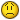# append multiple worksheets data into a single multi-dimensional array

N

#### noname

hi,

i have workbook which has columnar data in it which spans multiple sheets. i.e. each row is a single record which spans multiple worksheets. the rows are of fixed numbers across the sheets (say 200), but the columns vary to 255 or less. what i am trying to do is append the data in each sheet into a single multi-dimensional array (2-dimensions).

now the challenge is, i don't want to loop thru all the rows and columns ina sheet and go on appending at the end of the array.

has someone done something like this before using VBA? if so, can someone help? what will be a fast method?

Option Explicit

Sub ParseSheetsIntoSingleArray(UserWBK)
Dim wb As Workbook
Dim wk As Worksheet
Dim vfile() As Variant
Dim rng As Range
Dim counter As Integer
Dim numrows As Long, numcols As Long

Application.ScreenUpdating = False
Application.Calculation = xlCalculationManual

Set wb = Application.Workbooks.Open(UserWBK)

counter = 1

If Not wb Is Nothing Then
numrows = wb.Sheets(1).Cells(1, 1).End(xlDown).Row
ReDim vfile(1 To numrows, 1 To 1)

For Each wk In wb.Sheets
numrows = wk.Cells(1, 1).End(xlDown).Row
numcols = wk.Cells(1, wk.Columns.Count).End(xlToLeft).Column

ReDim Preserve vfile(1 To numrows, 1 To UBound(vfile, 2) + numcols)

If counter = 1 Then
Set rng = wk.Cells(1, 1).CurrentRegion
Else
'exclude 1st 2 columns in subsequent sheets
Set rng = wk.Range(wk.Cells(1, 3), wk.Cells(numrows, numcols))
End If

'i am assigning it this way, but in next iteration previous values dissapear
vfile = rng
counter = counter + 1

Next wk

wb.Close False
End If

Application.Calculation = xlCalculationAutomatic
Application.ScreenUpdating = True

End Sub

M

#### merjet

'i am assigning it this way, but in next iteration
'previous values dissappear
vfile = rng

Yes, because you are replacing, not appending.

I don't know how to append chunks (versus element by element) but guess that it would not reduce run-time anyway.

N

#### noname

Yes, because you are replacing, not appending.

I don't know how to append chunks (versus element by element) but guess that it would not reduce run-time anyway.

so i guess there isn't much in VBA to appending chunks of data to an array without the process of looping.....consider the case when the data flows thousands of rows down & many columns across. it would really be a slow process to append such data to an array using a loop.R

#### rumkus

why don't you dump your data on a temp sheet
and use filter or find method ?

I use below subroutine, for instance, to merge 20k+ rows.
It takes me no more than a sec really.

Sub trf(Sheet1 As String, Sheet2 As String)
Dim rng As Range
Dim lr As Double
With Worksheets(Sheet1)
Set rng = .Range("a2", .Range("a" & Rows.Count).End(xlUp))
End With
rng.EntireRow.Cut
lr = Worksheets(Sheet2).Range("A" & Rows.Count).End(xlUp).Offset(1,
0).Row
With Worksheets(Sheet2).Cells(lr, 1)
..Insert Shift:=xlDown
End With
End Sub

N

#### noname

why don't you dump your data on a temp sheet
and use filter or find method ?

I use below subroutine, for instance, to merge 20k+ rows.
It takes me no more than a sec really.

Sub trf(Sheet1 As String, Sheet2 As String)
Dim rng As Range
Dim lr As Double
With Worksheets(Sheet1)
Set rng = .Range("a2", .Range("a" & Rows.Count).End(xlUp))
End With
rng.EntireRow.Cut
lr = Worksheets(Sheet2).Range("A" & Rows.Count).End(xlUp).Offset(1,
0).Row
With Worksheets(Sheet2).Cells(lr, 1)
.Insert Shift:=xlDown
End With
End Sub

Hi,

the reason why i cannot dump into a sheet is because for a single record (row), the fields (columns) are spread across multiple sheets. 2ndly, working with worksheet would be slow if the records range into 1000 of rows.